# Double-Major Co. has a cost of equity of 11.7 percent and an aftertax cost of debt...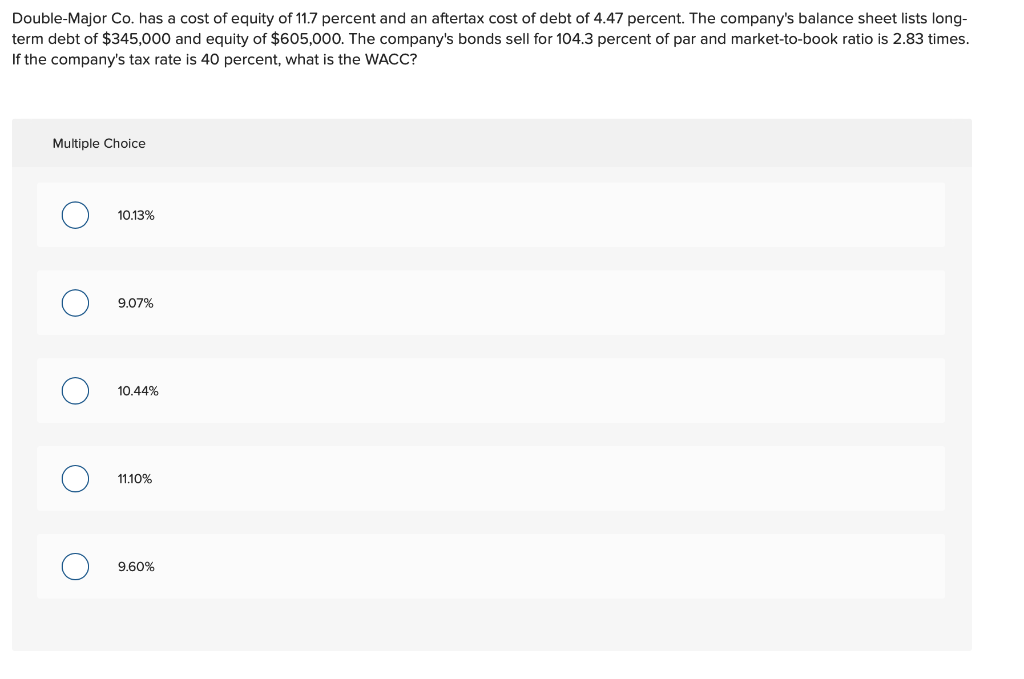Double-Major Co. has a cost of equity of 11.7 percent and an aftertax cost of debt of 4.47 percent. The company's balance sheet lists long- term debt of $345,000 and equity of$605,000. The company's bonds sell for 104.3 percent of par and market-to-book ratio is 2.83 times. If the company's tax rate is 40 percent, what is the WACC? Multiple Choice Ο 10.13% Ο 9.07% Ο 10.44% Ο 11.10% Ο 9.60%

Market to book ratio = Market value / book value

2.83 = Market value / 605,000

Market value = 1,712,150

Market value of debt = 104.3% of 345,000 = 359,835

Total market value = 1,712,150 + 359,835 = 2,071,985

WACC = Weights * costs

WACC = (359,835 / 2,071,985)*0.0447 + (1,712,150 / 2,071,985)*0.117

WACC = 0.007763 + 0.096681

WACC = 0.1044 or 10.44%

##### Add Answer to: Double-Major Co. has a cost of equity of 11.7 percent and an aftertax cost of debt...
Similar Homework Help Questions
• ### Upton Umbrellas has a cost of equity of 12.7 percent, the YTM on the company's bonds...

Upton Umbrellas has a cost of equity of 12.7 percent, the YTM on the company's bonds is 5.6 percent, and the tax rate is 40 percent. The company's bonds sell for 104.3 percent of par. The debt has a book value of $441,000 and total assets have a book value of$963,000. If the market-to-book ratio is 3.07 times, what is the company's WACC? Multiple Choice 10.62% 10.37% 8.49% 5.44% 8.33%

• ### Upton Umbrellas has a cost of equity of 11.2 percent, the YTM on the company's bonds...

Upton Umbrellas has a cost of equity of 11.2 percent, the YTM on the company's bonds is 5.8 percent, and the tax rate is 39 percent. The company's bonds sell for 93.2 percent of par. The debt has a book value of $396,000 and total assets have a book value of$948,000. If the market-to-book ratio is 2.62 times, what is the company's WACC?

• ### Upton Umbrellas has a cost of equity of 12.1 percent, the YTM on the company's bonds...

Upton Umbrellas has a cost of equity of 12.1 percent, the YTM on the company's bonds is 6.2 percent, and the tax rate is 39 percent. The company's bonds sell for 103.7 percent of par. The debt has a book value of $423,000 and total assets have a book value of$957,000. If the market-to-book ratio is 2.89 times, what is the company's WACC?

• ### Wentworth's Five and Dime Store has a cost of equity of 11.2 percent. The company has an aftertax cost of debt of 4.8 pe...

Wentworth's Five and Dime Store has a cost of equity of 11.2 percent. The company has an aftertax cost of debt of 4.8 percent, and the tax rate is 39 percent. If the company's debt–equity ratio is .72, what is the weighted average cost of capital? 6.39% 7.30% 8.52% 6.69% 7.74%

• ### Upton Umbrellas has a cost of equity of 11.5 percent, the YTM on the company's bonds is 6.1 percent, and the tax rate i...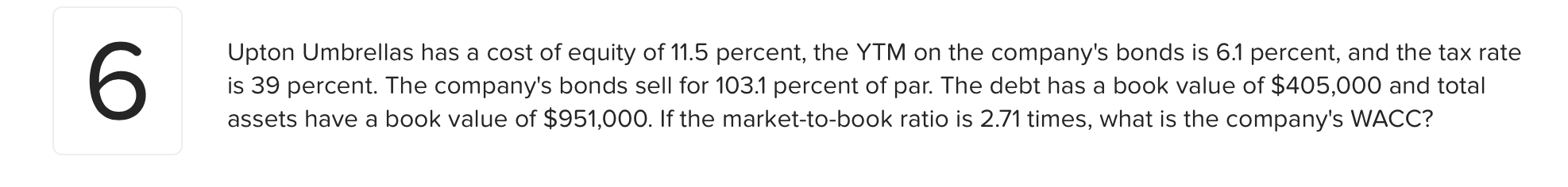Upton Umbrellas has a cost of equity of 11.5 percent, the YTM on the company's bonds is 6.1 percent, and the tax rate is 39 percent. The company's bonds sell for 103.1 percent of par. The debt has a book value of $405,000 and total assets have a book value of$951,000. If the market-to-book ratio is 2.71 times, what is the company's WACC?

• ### Upton Umbrellas has a cost of equity of 12.1 percent, the YTM on the company's bonds...

Upton Umbrellas has a cost of equity of 12.1 percent, the YTM on the company's bonds is 6.2 percent, and the tax rate is 39 percent. The company's bonds sell for 103.7 percent of par. The debt has a book value of $423,000 and total assets have a book value of$957,000. If the market-to-book ratio is 2.89 times, what is the company's WACC? 9.96% 5.62% 8.49% 10.26% 8.35%

• ### Upton Umbrellas has a cost of equity of 11.5 percent, the YTM on the company's bonds...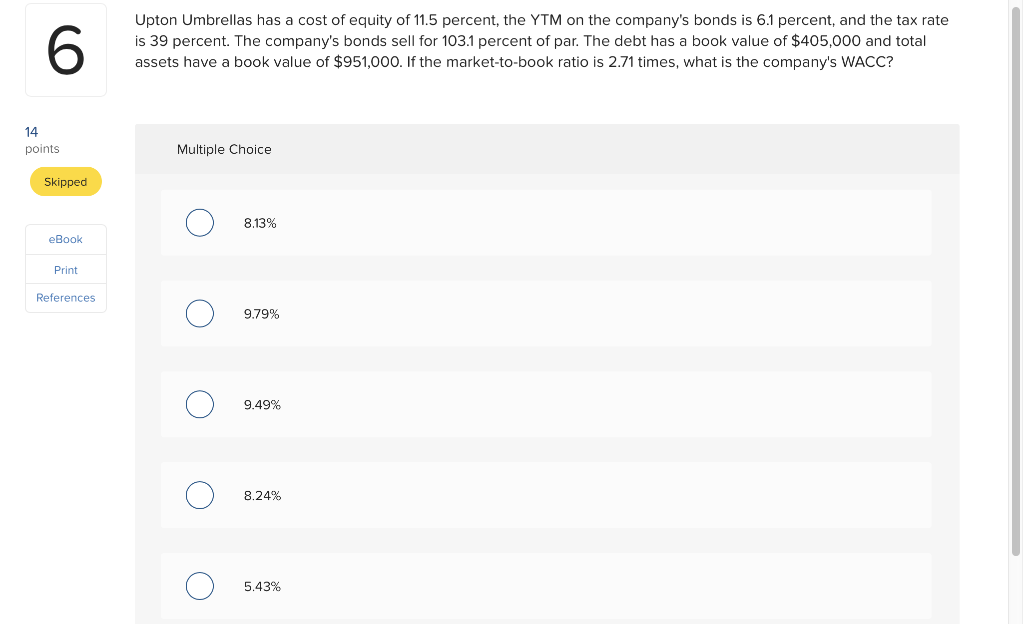Upton Umbrellas has a cost of equity of 11.5 percent, the YTM on the company's bonds is 6.1 percent, and the tax rate is 39 percent. The company's bonds sell for 103.1 percent of par. The debt has a book value of $405,000 and total assets have a book value of$951,000. If the market-to-book ratio is 2.71 times, what is the company's WACC? 6 14 points Multiple Choice Skipped 8.13% eBook Print References 9.79% 9.49% 8.24% 5.43%

• ### Take It All Away has a cost of equity of 10.45 percent, a pretax cost of debt of 5.21 percent, and a tax rate of 34 per...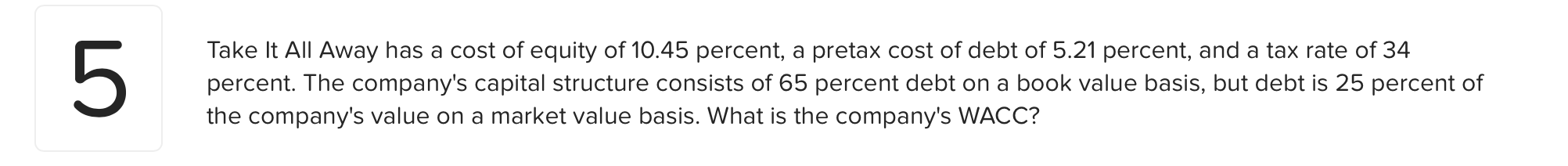Take It All Away has a cost of equity of 10.45 percent, a pretax cost of debt of 5.21 percent, and a tax rate of 34 percent. The company's capital structure consists of 65 percent debt on a book value basis, but debt is 25 percent of the company's value on a market value basis. What is the company's WACC?

• ### A firm has a cost of debt of 6.2 percent and a cost of equity of...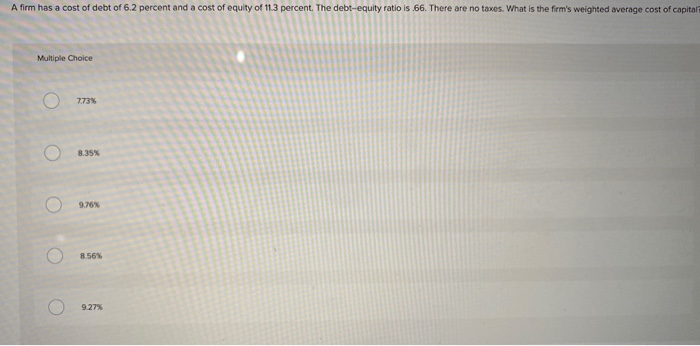A firm has a cost of debt of 6.2 percent and a cost of equity of 11.3 percent. The debt-equity ratio is 66. There are no taxes. What is the firm's weighted average cost of capital Multiple Choice Ο Ο Ο Ο Ο

• ### Evans Technology has the following capital structure. 35% Debt Common equity The aftertax cost of debt...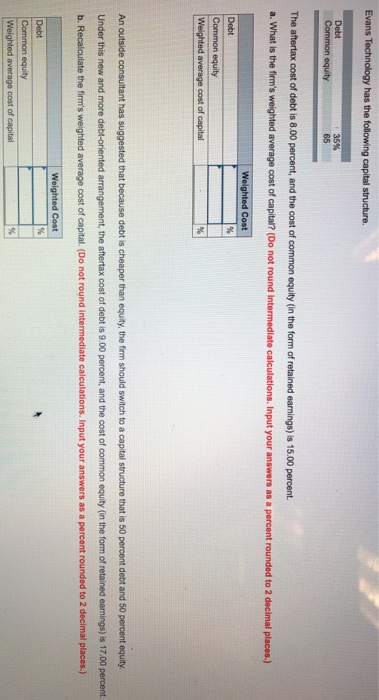Evans Technology has the following capital structure. 35% Debt Common equity The aftertax cost of debt is 8.00 percent, and the cost of common equity (in the form of retained earnings) is 15.00 percent. a. What is the firm's weighted average cost of capital? (Do not round Intermediate calculations. Input your answers as a percent rounded to 2 decimal places.) Weighted Cost Debt Common equity Weighted average cost of capital An outside consultant has suggested that because debt is cheaper...

Free Homework App